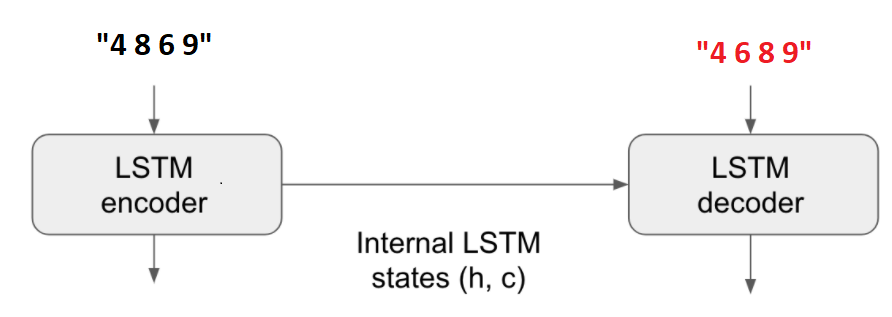# Combinatorial Optimization: From Supervised Learning to Reinforcement Learning – Part 1

xgb = XGBRegressor( objective=‘reg:squarederror’,
max_depth=10,
tree_method=‘gpu_hist’)
multioutputregressor = MultiOutputRegressor(xgb).fit(x_train, y_train)

```from numpy import asarray
from keras.models import Sequential
from keras.layers import Dense

# get the model
def get_model(n_inputs, n_outputs):
model = Sequential()
return model

n_inputs, n_outputs = x_train.shape, y_train.shape
# get model
model = get_model(n_inputs, n_outputs)
# fit the model on all data
model.fit(x_train, y_train, verbose=1, epochs=100)```

After training, we generate the test set with 200 samples, and calculate the accuracy of each model.

# 3. Sequence to sequence

A more natural approach is that if we take the input as a sequence, and the output is another sequence, we can use sequence to sequence models for this problem.I use a simple SeqtoSeq model for training.

```from numpy import array
from numpy import argmax
from numpy import array_equal
from keras.utils import to_categorical
from keras.models import Model
from keras.layers import Input
from keras.layers import LSTM
from keras.layers import Dense

# returns train, inference_encoder and inference_decoder models
def define_models(n_input, n_output, n_units):
# define training encoder
encoder_inputs = Input(shape=(None, n_input))
encoder = LSTM(n_units, return_state=True)
encoder_outputs, state_h, state_c = encoder(encoder_inputs)
encoder_states = [state_h, state_c]
# define training decoder
decoder_inputs = Input(shape=(None, n_output))
decoder_lstm = LSTM(n_units, return_sequences=True, return_state=True)
decoder_outputs, _, _ = decoder_lstm(decoder_inputs, initial_state=encoder_states)
decoder_dense = Dense(n_output, activation='softmax')
decoder_outputs = decoder_dense(decoder_outputs)
model = Model([encoder_inputs, decoder_inputs], decoder_outputs)
# define inference encoder
encoder_model = Model(encoder_inputs, encoder_states)
# define inference decoder
decoder_state_input_h = Input(shape=(n_units,))
decoder_state_input_c = Input(shape=(n_units,))
decoder_states_inputs = [decoder_state_input_h, decoder_state_input_c]
decoder_outputs, state_h, state_c = decoder_lstm(decoder_inputs, initial_state=decoder_states_inputs)
decoder_states = [state_h, state_c]
decoder_outputs = decoder_dense(decoder_outputs)
decoder_model = Model([decoder_inputs] + decoder_states_inputs, [decoder_outputs] + decoder_states)
# return all models
return model, encoder_model, decoder_model

# generate target given source sequence
def predict_sequence(infenc, infdec, source, n_steps, cardinality):
# encode
state = infenc.predict(source)
# start of sequence input
target_seq = array([0.0 for _ in range(cardinality)]).reshape(1, 1, cardinality)
# collect predictions
output = list()
for t in range(n_steps):
# predict next char
yhat, h, c = infdec.predict([target_seq] + state)
# store prediction
output.append(yhat[0,0,:])
# update state
state = [h, c]
# update target sequence
target_seq = yhat
return array(output)

# decode a one hot encoded string
def one_hot_decode(encoded_seq):
return [argmax(vector) for vector in encoded_seq]```

# 4. Result

 Model Training size (sample) Training time(s) Predict time(s) Accuracy XGB 1000 11.81 1.49 0.42 FCNNs 7.28 6.03 0.54 SeqtoSeq 7.14 (30 epochs) 80.3 0.97 XGB 5000 17.45 2.04 0.76 FCNNs 29.4 6.07 0.7 SeqtoSeq 10.9 (10 epochs) 80.3 1 XGB 50000 20.7 2.3 0.985 FCNNs 278.8 6.09 0.975 SeqtoSeq 11 (3 epochs) 79.1 1

( Run with Colab GPU)

All 3 models are capable of very good list sorting when there are large amount of training data. The SeqtoSeq approach gives the best accuracy. However, the predictable time of seq to seq seems to be longer than the other two options.

FCNNs and XGB models seem to give low accuracy when the training data set is small compare to SeqtoSeq model. One possible explanation is that in SeqtoSeq model, the model can learn the relationship between the number in the sequence. While in the XGB and FCNNs models, the tabular setting, make the relationship between each number in the table seems more independent. Therefore, more data are required to learn the relationship between numbers in the table.

# Reference

 Combinatorial optimization: https://en.wikipedia.org/wiki/Combinatorial_optimization